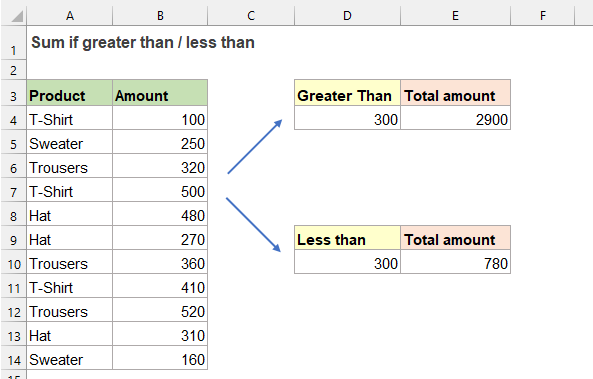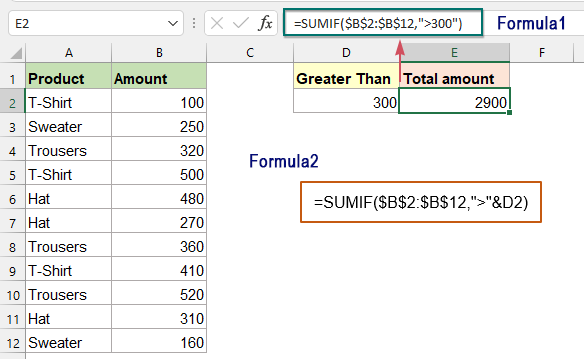## Sum if greater than or less than a specific value in Excel

To sum a list of values that are greater than or less than a specific value, the normal SUMIF function can help you to deal with this task. In this article, I will talk about how to use the SUMIF function to finish this problem in Excel.#### Sum if greater than or less than a specific value with SUMIF function

To sum values which are greater than or less than a given number, the generic syntaxes are:

Generic formula with hardcoded value:

Sum values greater than:      =SUMIF(range, ">value")
Sum values less than:           =SUMIF(range, "<value")
• range: The range of cells with values to be evaluated and summed;
• ">value", "<value": The criteria that is used to determine which of the cells should be summed. Here, indicates greater than or less than a specific value. (A variety of logical operators can be used to your needs, such as “=”, “>”, “>=”, “<”, “<=” etc.)

Generic formula with cell reference:

Sum values greater than:      =SUMIF(range, ">"& cell_ref)
Sum values less than:           =SUMIF(range, "<"& cell_ref)
• range: The range of cells with values to be evaluated and summed;
• ">value", "<value": The criteria that is used to determine which of the cells should be summed. Here, indicates greater than or less than a specific value. (A variety of logical operators can be used to your needs, such as “=”, “>”, “>=”, “<”, “<=” etc.)
• cell_ref: The cell contains the specific number that you want to sum values based on.

Take the above screenshot data as an example, I want to sum all the amount values that are greater than 300, please apply any one of the below formulas you like, and then press Enter key to get the result:

=SUMIF(\$B\$2:\$B\$12,">300")              (Type the criteria manually)
=SUMIF(\$B\$2:\$B\$12,">"&D2)
(Use a cell reference)Tips:

To sum up all amounts that are less than 300, please apply the following formulas:

=SUMIF(\$B\$2:\$B\$12,"<300")              (Type the criteria manually)
=SUMIF(\$B\$2:\$B\$12,"<"&D2)
(Use a cell reference)

#### Relative function used:

• SUMIF:
• The SUMIF function can help to sum cells based on one criterion.

#### More articles:

• Sum If Equal To One Of Many Things In Excel
• It may be easy for us to sum values based on a given criteria by using the SUMIF function. But, sometimes, you may need to sum values based on a list of items. For example, I have a data range which products are listed in Column A, and the corresponding sale amounts are listed in Column B. Now, I want to get the total amount based on the listed products in range D4:D6 as below screenshot shown. How to solve this problem quickly and easily in Excel?
• Sum Smallest Or Bottom N Values In Excel
• In Excel, it is easy for us to sum a range of cells by using the SUM function. Sometimes, you may need to sum the smallest or bottom 3, 5 or n numbers in a data range as below screenshot shown. In this case, the SUMPRODUCT together with the SMALL function can help you to solve this problem in Excel.
• Sum If Between Two Values In Excel
• In your daily work, it may be common for you to calculate total score or total amount for a range. To solve this problem, you can use the SUMIFS function in Excel. The SUMIFS function is used to sum up particular cells based on multiple criteria. This tutorial will demonstrate how to use the SUMIFS function to sum data between two numbers.

### The Best Office Productivity Tools

#### Kutools for Excel - Helps You To Stand Out From Crowd

 Popular Features: Find/Highlight/Identify Duplicates  |  Delete Blank Rows  |  Combine Columns or Cells without Losing Data  |  Round without Formula ... Super VLookup: Multiple Criteria  |  Multiple Value  |  Across Multi-Sheets  |  Fuzzy Lookup... Adv. Drop-down List: Easy Drop Down List  |  Dependent Drop Down List  |  Multi-select Drop Down List... Column Manager: Add a Specific Number of Columns  |  Move Columns  |  Toggle Visibility Status of Hidden Columns  |  Compare Columns to Select Same & Different Cells ... Featured Features: Grid Focus  |  Design View  |  Big Formula Bar  |  Workbook & Sheet Manager | Resource Library (Auto Text)  |  Date Picker  |  Combine Worksheets  |  Encrypt/Decrypt Cells  |  Send Emails by List  |  Super Filter  |  Special Filter (filter bold/italic/strikethrough...) ... Top 15 Toolsets:  12 Text Tools (Add Text, Remove Characters ...)  |  50+ Chart Types (Gantt Chart ...)  |  40+ Practical Formulas (Calculate age based on birthday ...)  |  19 Insertion Tools (Insert QR Code, Insert Picture from Path ...)  |  12 Conversion Tools (Numbers to Words, Currency Conversion ...)  |  7 Merge & Split Tools (Advanced Combine Rows, Split Cells ...)  |  ... and more

Kutools for Excel Boasts Over 300 Features, Ensuring That What You Need is Just A Click Away...

Supports Office/Excel 2007-2021 and 365   |   Available in 44 Languages   |   Easy to Uninstall Completely#### Office Tab - Enable Tabbed Reading and Editing in Microsoft Office (include Excel)

• One second to switch between dozens of open documents!
• Reduce hundreds of mouse clicks for you every day, say goodbye to mouse hand.
• Increases your productivity by 50% when viewing and editing multiple documents.
• Brings Efficient Tabs to Office (include Excel), Just Like Chrome, Edge and Firefox.No ratings yet. Be the first to rate!
This comment was minimized by the moderator on the site
Comment Trouver Toutes Les Combinaisons Qui Égalent Une Somme Donnée Dans Excel?
6 nombres de 1 à 18 qui font un total supérieur ou égal à un nombre dans l'intervalle [50-60]
merci de m'aider
This comment was minimized by the moderator on the site
Hello, leslie jean
At present, there's no good way to solve this task, but if you want to find all combinations that are equal to a given sum, rather than in an interval, please view this article:
How To Find All Combinations That Equal A Given Sum In Excel?
Thank you!
There are no comments posted here yet